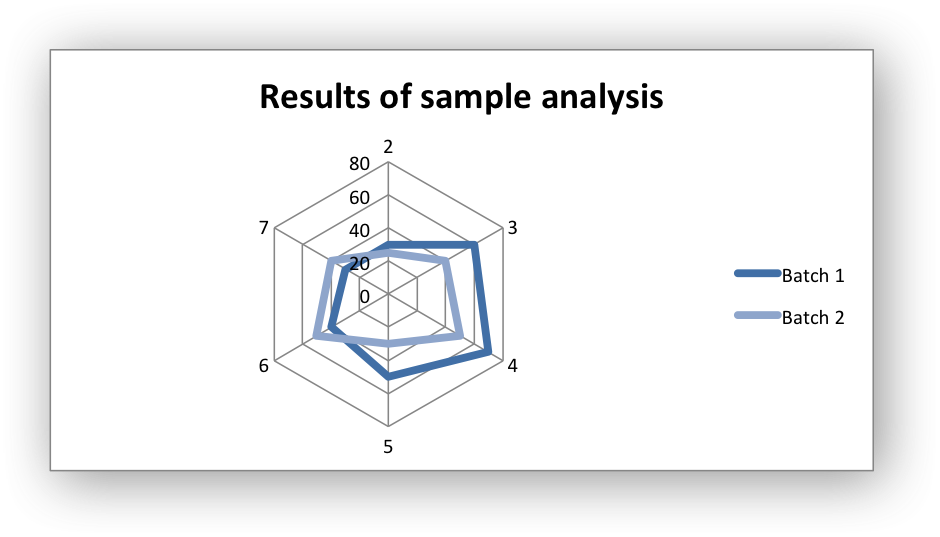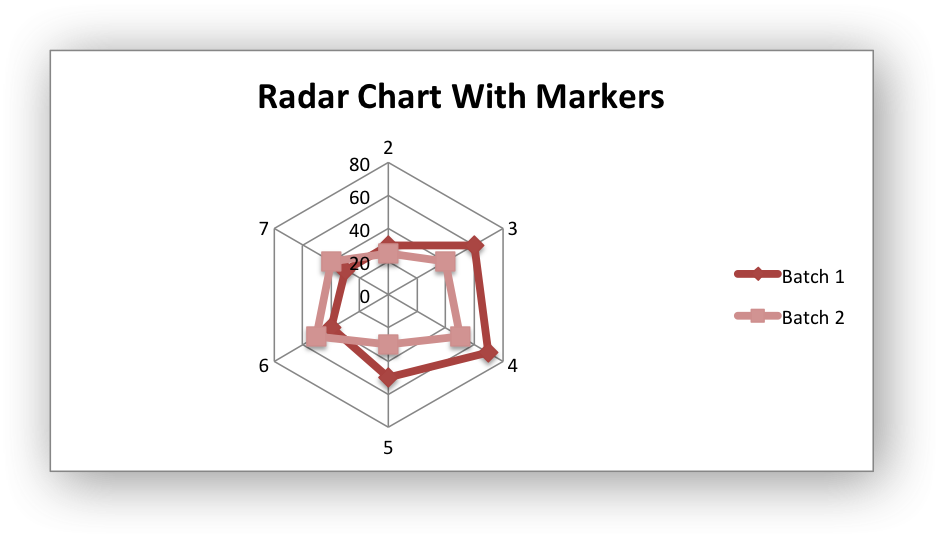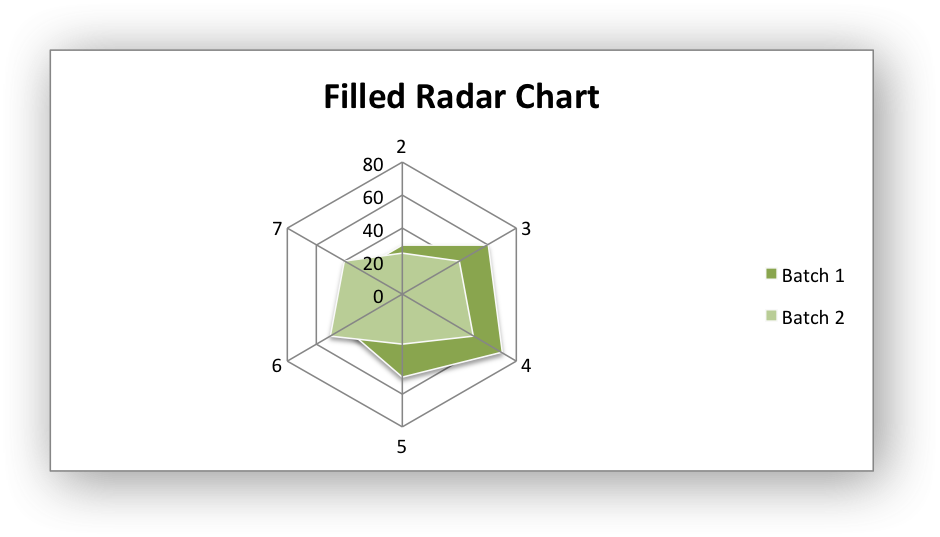Example of creating Excel Column charts.

Chart 1 in the following example is a default radar chart:Chart 2 in the following example is a radar chart with markers:Chart 3 in the following example is a filled radar chart:```#######################################################################
#
# An example of creating Excel Radar charts with Python and XlsxWriter.
#
# Copyright 2013-2023, John McNamara, jmcnamara@cpan.org
#
import xlsxwriter

# Add the worksheet data that the charts will refer to.
headings = ["Number", "Batch 1", "Batch 2"]
data = [
[2, 3, 4, 5, 6, 7],
[30, 60, 70, 50, 40, 30],
[25, 40, 50, 30, 50, 40],
]

worksheet.write_column("A2", data)
worksheet.write_column("B2", data)
worksheet.write_column("C2", data)

#######################################################################
#
# Create a new radar chart.
#

# Configure the first series.
{
"name": "=Sheet1!\$B\$1",
"categories": "=Sheet1!\$A\$2:\$A\$7",
"values": "=Sheet1!\$B\$2:\$B\$7",
}
)

# Configure second series. Note use of alternative syntax to define ranges.
{
"name": ["Sheet1", 0, 2],
"categories": ["Sheet1", 1, 0, 6, 0],
"values": ["Sheet1", 1, 2, 6, 2],
}
)

chart1.set_title({"name": "Results of sample analysis"})

# Set an Excel chart style.
chart1.set_style(11)

# Insert the chart into the worksheet (with an offset).
worksheet.insert_chart("D2", chart1, {"x_offset": 25, "y_offset": 10})

#######################################################################
#
# Create a radar chart with markers chart sub-type.
#

# Configure the first series.
{
"name": "=Sheet1!\$B\$1",
"categories": "=Sheet1!\$A\$2:\$A\$7",
"values": "=Sheet1!\$B\$2:\$B\$7",
}
)

# Configure second series.
{
"name": "=Sheet1!\$C\$1",
"categories": "=Sheet1!\$A\$2:\$A\$7",
"values": "=Sheet1!\$C\$2:\$C\$7",
}
)

# Set an Excel chart style.
chart2.set_style(12)

# Insert the chart into the worksheet (with an offset).
worksheet.insert_chart("D18", chart2, {"x_offset": 25, "y_offset": 10})

#######################################################################
#
# Create a filled radar chart sub-type.
#

# Configure the first series.
{
"name": "=Sheet1!\$B\$1",
"categories": "=Sheet1!\$A\$2:\$A\$7",
"values": "=Sheet1!\$B\$2:\$B\$7",
}
)

# Configure second series.
{
"name": "=Sheet1!\$C\$1",
"categories": "=Sheet1!\$A\$2:\$A\$7",
"values": "=Sheet1!\$C\$2:\$C\$7",
}
)